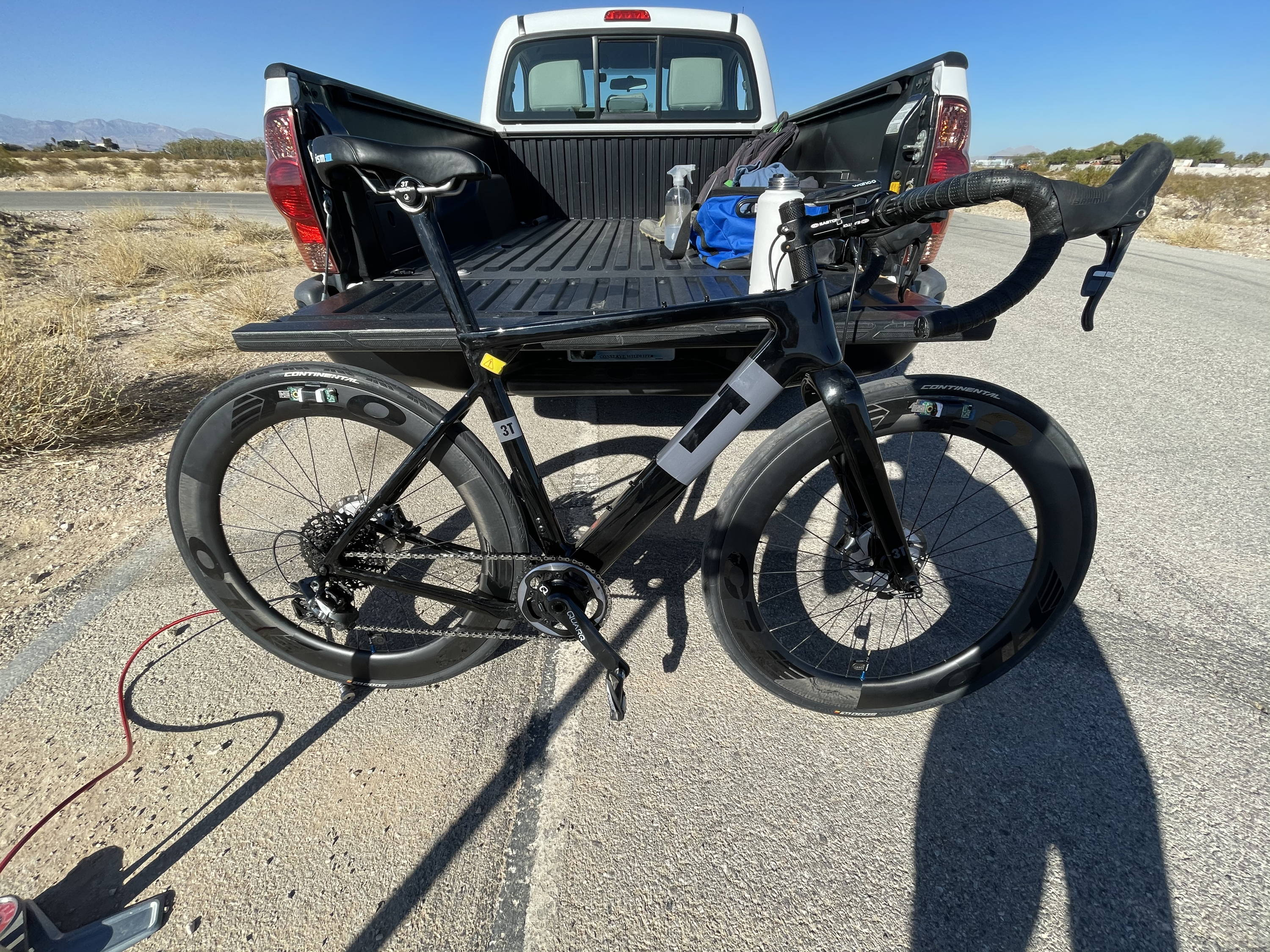# Measuring Wheel Vibration – Speed Is Key

The last time we wrote about vibration we talked about our work with rollers. We were noting how the rollers showed the same FFT graph shape, but the excitation was not the same as when tested on the road. For a refresher on this check out our last post here.

Based on all of our vibration work to date, we determined that the best way to find an impedance break point was to develop an algorithm that would calculate the amount of energy produced by a wheel and tire combination at various tire pressures. Our previous work showed that as the tire pressure increased, the energy of the system increased as well. If we could plot this successfully, our belief was that we would see an increase in the slope of a line that plotted tire pressure vs wheel energy at the impedance break point.## The Test

To test this theory, we mounted two 3D Accelerometers to a set of FLO 64 AS wheels and tested four pressures so that we could plot the total wheel energy vs. tire pressure. To be thorough, we had two riders run the same course.

We rode the same course to collect the data. The out and back loop had small grade variations so we did not expect to see anything drastic. However, drastic is what we saw. After running the tests we plotted the data and nothing made sense. Higher pressure had lower energy than lower pressures on some tests and vice versa on others. This made no sense and showed no repeatability.

## What Happened?

It took us a while to determine what happened during the testing to produce such drastically different results. Here is what we determined.

1. The algorithm was sampling data from 20 second intervals on the course. This sampling was not in the same course location on the out and back.
2. The slight variation in grade produced a slightly different rider speed.
3. Our theory was that the difference in rider speed could have impacted the overall energy results of the tests. Our initial test held power constant.

## Holding Speed Steady

To test our theory that speed may have an impact on overall wheel energy we conducted the following test:

1. We would hold the pressure constant.
2. We would test four different speeds.
3. We would use two different riders to see if we could repeat the results.

If our theory was true, we would notice a difference in overall wheel energy as rider speed changed and pressure remained the same.

## The Results

We were quite shocked when we saw the results. The energy difference was much more extreme than we imagined. The graphs below shows the four different speeds (roughly 12mph, 15mph, 18mph, and 21mph) and the associated wheel energy of a front and rear FLO 64 AS wheel.

What is really cool to see is the beauty of the graphed data. In all the years we’ve collected and plotted data to understand what is happening in the real world, I always love when data produces a clean result. In an engineering way, it’s beautiful.

Now we do not have enough info to draw an exact conclusion, but what you see here is potentially the following:

1. The lower speeds produce a liner increase in wheel energy.
2. At 18mph to 21mph you see an increase in the slope, which appears to indicate the pressure tested incurred an impedance break point between 18 and 21mph.

## What This Means

Here is what we now know from our data on wheel energy as speed increases.

1. Impedance break points are not related solely to pressure, they produce a matrix of values related to speed, surface roughness, rider weight, and pressure.
2. Velocity is much more of a factor than we originally thought.
3. Tire pressure recommendations are more like a finger print than a simple table.

## Next Steps

Our next step is to run a test that holds two constant speeds – a high speed and a low speed -, and to test a number of tire pressures. The test will use pressures from 60-120psi in 5psi increments. Stay tuned for the results.

WHEELS